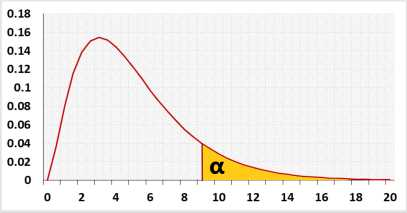# Kaplan Meier Survival Analysis

Draws the Kaplan-Meier plot and calculates the log-rank test (log rank test is only for two group).

## Test calculation

Right-tailed For the Goodness of Fit Test, you can use only the right tail test.
The population's name
Significance level (0-1), maximum chance allowed rejecting H0 while H0 is correct (Type1 Error)
The number of estimated variables.
Degree Of Freedom: df = k - m - 1
The ratio of the mean difference, that the test needs to be able to identify and the standard deviation. For example, 0.1 small, 0.3 medium, 0.5 large.
The sample size is calculated from total events and censored events. If by the end of the research some subjects didn't have an event, please insert censored data for these subjects.

Enter raw data directly
Enter raw data from excel
Enter aggregate data
 Time Events Censors Group
Please fill in one row per subject. Event/Censored - 1 for event or 0 for censored event.
 TimeO Event/Censored Group

The tool will trim empty cells, spaces or non-numeric data in the numeric columns.
If you enter only one group the log-rank test will not run.
You may use more than two groups.

## Enter sample data

You may copy data from Excel, Google sheets or any tool that separate data with Tab and Line Feed.
Copy the data, one block of 3 consecutive columns includes the header, and paste below.
Copy the data,It is okay to leave empty cells, empty cells or non numeric cells won't be counted

## Log-rank test

Group
Observed
Frequency
*Expected
Frequencies
Summary
 k number of groups n sample size χ² Chi square test statistic
. (for log-rank test m=0).
validation message

## Information

Event: Maintenance failure, recovery, disease occurrence, death, etc.
Censored event: the main event didn't happen since the subject left the experiment before the ending time, or due to the termination of the experiment.

Hypotheses
H0: Model fits, equal survival distributions
H1: Model doesn't fit
Test statisticχ² distributionTarget: Check if the statistical model fits the observations.
The test uses Chi-square distribution.

The log-rank test model assumes the events per subject distributes evenly between the groups. The expected number of events is calculated per each time value.
Example with two groups A and B.
Expected value = nA(dA + dB)/(nA + nB)

The page was created per Anna P request.

## R Code

The following R code should produce the same results as the alternative log-rank test